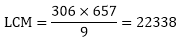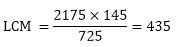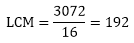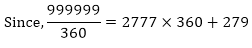×#### Thank you for registering.

One of our academic counsellors will contact you within 1 working day.

Click to Chat

1800-1023-196

+91-120-4616500

CART 0

• 0

MY CART (5)

Use Coupon: CART20 and get 20% off on all online Study Material

ITEM
DETAILS
MRP
DISCOUNT
FINAL PRICE
Total Price: Rs.

There are no items in this cart.
Continue Shopping• Complete JEE Main/Advanced Course and Test Series
• OFFERED PRICE: Rs. 15,900
• View Details

```Chapter 1: Real Numbers Exercise – 1.4

Question: 1

Find the LCM and HCF of the following pairs of integers and verify that LCM × HCF = product of the integers:

(i) 26 and 91

(ii) 510 and 92

(iii) 336 and 54

Solution:

To Find: LCM and HCF of following pairs of integers

To Verify: L.C.M × H.C.F = product of the numbers

(i) 26 and 91

Let us first find the factors of 26 and 91

26 = 2 × 13

91= 7 × 13

L.C.M of 26, and 91 = 2 × 7 × 13

L.C.M of 26, and 91 = 182

H.C.F of 26, and 91 = 182

We know that, L.C.M × H.C.F = First number × Second number

182 × 13 = 26 × 91 = 2366 = 2366

Hence verified

(ii) 510 and 92

Let us first find the factors of 510 and 92

510 = 2 × 3 × 5 × 17

92 = 2 × 2 × 23

L.C.M of 510 and 92 = 2 × 2 × 3 × 5 × 23 × 17

L.C.M of 510 and 92 = 23460

H.C.F of 510 and 92 = 2

We know that, L.C.M × H.C.F = First Number × Second Number

23460 × 2 = 510 × 92

46920 = 46920

Hence verified.

(iii)  336 and 54

Let us first find the factors of 336 and 54

336 = 2 × 2 × 2 × 2 × 3 × 3 × 3 × 7

54 = 2 × 3 × 3 ×3

L.C.M of 336 and 54 = 2 × 2 × 2 × 2 × 3 × 3 × 3 × 7

L.C.M of 336 and 54 = 3024

H.C.F of 336 and 54 = 6

We know that, L.C.M × H.C.F = First Number × Second Number

3024 × 6 = 336 × 54

18144 = 18144

Hence verified

Question: 2

Find the LCM and HCF of the following integers by applying the prime factorization method:

(i) 12, 15 and 21

(ii) 17, 23 and 29

(iii) 8, 9 and 25

(iv) 40, 36 and 126

(v) 84, 90 and 120

(vi) 24, 15 and 36

Solution:

To Find: LCM and HCF of following pairs of integers

(i) 15, 12 and 21

Let us first find the factors of 15, 12 and 21

12 = 2 × 2 × 3

15 = 3 × 5

21 = 3 × 7

L.C.M of 12, 15 and 21 = 2 × 2 × 3 × 5 × 7

L.C.M of 12, 15 and 21 = 420

H.C.F of 12, 15 and 21 = 3

(ii) 17, 23 and 29

Let us first find the factors of 17, 23 and 29

17 = 1 × 17

23 = 1 × 23

29 = 1 × 29

L.C.M of 17, 23 and 29 = 1 × 17 × 23 × 29

L.C.M of 17, 23 and 29 = 11339

H.C.F of 17, 23 and 29 = 1

(iii) 8, 9 and 25

Let us first find the factors of 8, 9 and 25

8 = 2 × 2 ×2

9 = 3 × 3

25 = 5 × 5

L.C.M of 8, 9 and 25 =23 × 32 × 52

L.C.M of 8, 9 and 25 = 1800

H.C.F of 8, 9 and 25 = 1

(iv) 40, 36 and 126

Let us first find the factors of 40, 36 and 126

40 = 23 × 5

36 = 23 × 32

126 = 2 × 3 × 3 × 7

L.C.M of 40, 36 and 126 = 23 × 32 × 5 × 7

L.C.M of 40, 36 and 126 = 2520

H.C.F of 40, 36 and 126 = 2

(v) 84, 90 and 120

Let us first find the factors of 84, 90 and 120

84 = 2 × 2 × 3 × 7

90 = 2 × 3 × 3 × 5

120 = 2 × 2 × 2 × 3 × 5

L.C.M of 84, 90 and 120 = 23 × 32 × 5 × 7

L.C.M of 84, 90 and 120 = 2520

H.C.F of 84, 90 and 120 = 6

(vi) 24, 15 and 36

Let us first find the factors of 24, 15 and 36

24 = 23 × 3

15 = 3 × 5

36 = 2 × 2 × 3 × 3

LCM of 24, 15 and 36 = 2 × 2 × 2 × 3 × 3 × 5

LCM of 24, 15 and 36 = 360

HCF of 24, 15 and 36 = 3

Question: 3

Given that HCF (306, 657) = 9, find LCM (306, 657 )

Solution:

Given:

HCF of two numbers 306 and 657 is 9

To find: LCM of number

We know that,

LCM × HCF = first number × second number

LCM × 9 = 306 × 657Question: 4

Can two numbers have 16 as their HCF and 380 as their as their LCM? Give reason.

Solution:

To find: can two numbers have 16 as their HCF and 380 as their LCM

On dividing 380 by 16 we get 23 as the quotient and 12 as the remainder

Since LCM is not exactly divisible by the HCF, two number cannot have 16 as their HCF and 380 as their

Question: 5

The HCF of two numbers is 145 and their LCM is 2175. If one number is 725, find the other.

Solution:

Given:

LCM and HCF of two numbers 145 and 2175 respectively.

If one number is 725

To find: other number

We know that,

LCM × HCF = first number × second number

2175 × 145 = 725 × second numberQuestion: 6

The HCF of two numbers is 16 and their LCM is 3072. Find the LCM.

Solution:

Given: HCF of two numbers is 16. If the product of the numbers is 3072

To find: LCM of number

We know that,

LCM × HCF = first number × second number

LCM × 16 = 3072Question: 7

The LCM and HCF of two numbers are 180 and 6 respectively. If one of the numbers is 30, find the other number.

Solution:

Given:

LCM and HCF of two numbers 180 and 6 respectively.

If one number is 30

To find: other number

We know that,

LCM × HCF = First Number × Second Number

180 × 6 = 30 × Second NumberQuestion: 8

Find the smallest number which when increased by 17 is exactly divisible by both 520 and 468.

Solution:

To Find Smallest number which when increased by 17 is exactly divisible by both 520 and 468. L.C.M OF 520 and 468

520 = 23 × 5 × 13

468 = 2 × 2 × 3 × 3 × 13

LCM of 520 and 468 = 23 × 32 × 5 × 13 = 4680

Hence 4680 is the least number which exactly divides 520 and 468  i.e. we will get a remainder of 0 in this case. But we need the Smallest number which when increased by 17 is exactly divided by 520 and 468.

Therefore = 4680 – 17 = 46631

Hence 4663 is Smallest number which when increased by 17 is exactly divisible by both 520 and 468.

Question: 9

Find the smallest number which leaves remainders 8 and 12 when divided by 28 and 32 respectively.

Solution:

To Find: The smallest number which leaves remainders 8 and 12 when divided by 28 and 32 respectively.

L.C.M of 28 and 32.

28 = 2 × 2 × 7

32 = 25

L.C.M of 28, and 32 = 25 × 7 = 224

Hence 224 is the least number which e×actly divides 28 and 32 i.e. we will get a remainder of 0 in this case. But we need the smallest number which leaves remainders 8 and 12 when divided by 28 and 32 respectively.

Therefore = 224 – 8 – 12 = 204

Hence 2041 is the smallest number which leaves remainders 8 and 12 when divided by 28 and 32 respectively

Question: 10

What is the smallest number that, when divided by 35, 56 and 91 leaves remainders of 7 in each case?

Solution:

To Find: Smallest number that. When divided by 35, 56 and 91 leaves remainder of 7 in each case L.C.M OF 35, 56 and 91

35 = 5 × 7

56 = 23 × 7

91 = 13 × 7

L.C.M of 35, 56 and 91 = 23 × 7 × 5 × 13 = 3640

Hence 84 is the least number which exactly divides 28, 42 and 84 i.e. we will get a remainder of 0 in this case. But we need the smallest number that, when divided by 35, 56 and 91 leaves remainder of 7 in each case.

Therefore = 3640 + 7 = 3647

Hence 36471 is smallest number that, when divided by 35, 56 and 91 leaves remainder of 7 in each case.

Question: 11

A rectangular courtyard is 18 m 72 cm long and 13 m 20 cm broad. It is to be paved with square tiles of the same size. Find the least possible number of such tiles.

Solution:

Given: A rectangular yard is 18 m 72 cm long and 13 m 20 cm broad .It is to be paved with square tiles of the same size.

TO FIND: Least possible number of such tiles.

Length of the yard = 18 m 72 cm = 1800 cm + 72 cm = 1872 cm (therefore, 1 m = 100 cm)

Breadth of the yard = 13 in 20 cm = 1300 cm + 20 cm = 1320 cm

The size of the square tile of same size needed to the pave the rectangular yard is equals the HCF of the length and breadth of the rectangular yard.

Prime factorization of 1872 = 24 × 32 × 13

Prime factorization of 1320 = 23 × 3 × 5 × 11

HCF of 1872 and 1320 = 23 × 3 = 24

Therefore, Length of side of the square tile = 24 cm

Number of tiles required = Area of the courtyard, Area of each tile = Length × Breadth

Side2 = 1872 cm × 1320 cm 24 cm = 4290.

Thus, the least possible number of tiles required is 4290.

Question: 12

Find the greatest number of 6 digits exactly divisible by 24, 15 and 36.

Solution:

To Find: Greatest number of 6 digits exactly divisible by 24, 15 and 36

The greatest 6 digit number be 999999 divisible by 24, 15 and 36

24 = 2 × 2 × 2 × 3

15 = 3 × 5

36 = 2 × 2 × 3 × 3

L.C.M of 24, 15 and 36 = 360Therefore, the remainder is 279. Hence the desired number is = 999999 – 279 = 9997201

Hence 9997201 is the greatest number of 6 digits exactly divisible by 24, 15 and 36.

Question: 13

Determine the number nearest to 110000 but greater 100000 which is exactly divisible by each of 8, 15 and 21.

Solution:

To Find: The number nearest to 110000 but greater than 100000 which is exactly divisible by each of 8, 15 and 21.

L.C.M of 8, 15 and 21.

8 = 2 × 2 × 2

15 = 3 × 5

21 = 3 × 7

L.C.M of 8, 15 and 21 =23 × 3 × 5 × 71= 840

When 110000 is divided by 840, the remainder is obtained as 800.

Now, 110000 — 800 = 109200 is divisible by each of 8, 15 and 21.

Also, 110000 + 40 = 110040 is divisible by each of 8, 15 and 21.

109200 and 110040 are greater than 100000.

Hence, 110040 is the number nearest to 110000 but greater than 100000 which is exactly divisible by each of 8, 15 and 21.

Question: 14

Find the least number that is divisible by all the numbers between 1 and 10 (both inclusive)

Solution:

To Find: Least number that is divisible by all the numbers between 1 and 10 (both inclusive) Let us first find the L.C.M of all the numbers between 1 and 10 (both inclusive)

1 = 1

2 = 2

3 = 3

4 = 2 × 2

5 = 5

6 = 2 × 3

7 = 7

8 = 2 × 2 × 2

9 = 3 × 3

10 = 2 × 5

L.C.M 2520

Hence 2520 is the least number that is divisible by all the numbers between 1 and 10 (both inclusive)

Question: 15

A circular field has a circumference of 360km. three cyclists start together and can cycle 48, 60 and 72 km a day, round the field. When will they meet again?

Solution:

Given: A circular field has a circumference of 360 km. Three cyclists start together and can cycle 48, 60, and 72 km a day, round the field.

TO FIND: When they meet again.

In order to calculate the time when they meet, we first find out the time taken by each cyclist in covering the distance.

Number of days 1st cyclist took to cover 360 km = Total distance covered in 1 day = 360/48 = 7.5 = 7510 = 152 days

Similarly, number of days taken by 2nd cyclist to cover same distance = 360/60 = 6 days

Also, number of days taken by 3rd cyclist to cover this distance = 360/72 = 5 days

Now, LCM of 152, 6 and 5 = LCM of numerators

HCF of denominators = 30/1 = 30 days

Thus, all of them will take 30 days to meet again.

Question: 16

In a morning walk three persons step off together, their steps measure 80cm, 85 cm and 90 cm respectively. What is the minimum distance each should walk so that he can cover the distance in complete steps?

Solution:

Given: In a morning walk, three persons step off together. Their steps measure 80 cm, 85 cm and 90 cm.

To Find minimum distance each should walk so that all can cover the same distance in complete steps.

The distance covered by each of them is required to be same as well as minimum. The required distance each should walk would be the L.C.M of the measures of their steps i.e. 80 cm, 85 cm, and 90 cm,

So we have to find the L.C.M of 80 cm, 85 cm, and 90 cm.

80 = 24 × 5

85 = 17 × 5

90 = 2 × 3 × 3 × 5

L.C.M of 80, 85 and 90 = 24 × 3 × 3 × 5 × 17 = 12240 cm

Hence minimum 12240 cm distance each should walk so that all can cove the same distance in complete steps.
```### Course Features

• 728 Video Lectures
• Revision Notes
• Previous Year Papers
• Mind Map
• Study Planner
• NCERT Solutions
• Discussion Forum
• Test paper with Video Solution